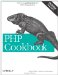# Recipe 2.4. Operating on a Series of Integers

#### 2.4.1. Problem

You want to apply a piece of code to a range of integers.

#### 2.4.2. Solution

Use a for loop:

`<?php for (\$i = \$start; \$i <= \$end; \$i++) {     plot_point(\$i); } ?>`

You can increment using values other than 1. For example:

`<?php for (\$i = \$start; \$i <= \$end; \$i += \$increment) {     plot_point(\$i); } ?>`

If you want to preserve the numbers for use beyond iteration, use the range( ) method:

`<?php \$range = range(\$start, \$end); ?>`

#### 2.4.3. Discussion

Loops like this are common. For instance, you could be plotting a function and need to calculate the results for multiple points on the graph. Or you could be a student counting down the number of seconds until the end of school.

The for loop method uses a single integer and you have great control over the loop, because you can increment and decrement \$i freely. Also, you can modify \$i from inside the loop.

In the last example in the Solution, range( ) returns an array with values from \$start to \$end. The advantage of using range( ) is its brevity, but this technique has a few disadvantages. For one, a large array can take up unnecessary memory. Also, you're forced to increment the series one number at a time, so you can't loop through a series of even integers, for example.

It's valid for \$start to be larger than \$end. In this case, the numbers returned by range( ) are in descending order. Also, you can use it to retrieve character sequences:

`<?php print_r(range('l', 'p')); ?> Array (      => l      => m      => n      => o      => p )`

Recipe 4.3 for details on initializing an array to a range of integers; documentation on range( ) at http://www.php.net/range.PHP Cookbook: Solutions and Examples for PHP Programmers
ISBN: 0596101015
EAN: 2147483647
Year: 2006
Pages: 445

Similar book on Amazon